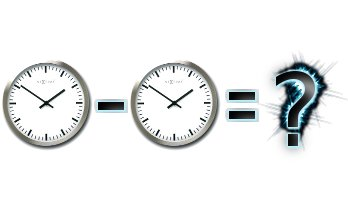# Subtract two datesIf you've asked the following: How to calculate the difference between two dates? , What is the time between two dates? or How subtract dates?, we have an answer for you. On this page you will know the difference between any two dates in terms of seconds, minutes, hours or days. It's easy, just change the dates shown below, the calculation is displayed immediately:

Difference Between Two Dates